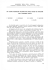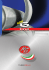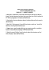R

Transcription

R
Solutions to FE Problems
Chapter 2
2FE-1 What is the power generated by the source in the network in Fig. 2PFE-1?
a
RS
R2
R1
120V
c
b
R3
R4
R5
Resistors R1, R2, and R3 are connected in delta. A delta-wye transformation can be conducted.
R1 R2
6k (18k )
Ra =
=
= 3kΩ
R1 + R2 + R3 6k + 18k + 12k
R2 R3
18k (12k )
=
= 6kΩ
R1 + R2 + R3 6k + 18k + 12k
R1 R3
6k (12k )
Rc =
=
= 2kΩ
R1 + R2 + R3 6k + 18k + 12k
Rb =
a
RS
Ra
120V
Rc
Rb
c
b
R4
Req = Rs + Ra + [( Rc + R4 ) ( Rb + R5 )] = 5k + 3k + [(2k + 4k ) (6k + 6k )]
6k (12k )
= 12kΩ
6k + 12k
(120) 2
=
= 1.2W
12k
Req = 8k +
PVs =
Vs2
Req
2FE-2 Find Vab in the circuit in Fig. 2PFE-2.
I1
10Ω
b
15Ω
I2
10Ω
a
4A
5Ω
R5
10 + 5


I1 = 
( 4 ) = 1.5 A
 10 + 5 + 10 + 15 
10 + 15


I2 = 
( 4 ) = 2.5 A
 10 + 5 + 10 + 15 
V5Ω = 5(2.5) = 12.5V
V15Ω = 15(1.5) = 22.5V
Vab = 12.5 − 22.5 = −10V
2FE-3 If Req=10.8Ω in the circuit in Fig. 2PFE-3, what is R2?
Req = ( R2 8) + 4 + 2
( R2 )8
+6
R2 + 8
8 R2
10.8 − 6 =
R2 + 8
3.2 R2 = 38.4
R2 = 12Ω
Req =
2FE-4 Find the equivalent resistance of the circuit in Fig. 2PFE-4 at the terminals A-B.
R1
R6
R2
R3
R5
R AB
R7
R4
R AB = { [[( R6 + R7 )
R5 ] + R4 ]
R AB = { [[24k 12k ] + 4k ]
R3 } + ( R1 R2 )
6k } + (12k 6k )
  24k (12k )
  12k (6k ) 

R AB =  
+ 4k  6 k  + 


  24k + 12k
  12k + 6k 
R AB = (12k 6k ) + 4k =
12k (6k )
+ 4k
12k + 6k
R AB = 8kΩ
2FE-5 The 100V source is absorbing 50W of power in the network in Fig. 2PFE-5. What is R?
A
10Ω
I1 10Ω
I2
R
100V
5A
200V
P = VI 1
50 = −100 I 1
I 1 = −0.5 A
KVL around the right loop:
100 + I 2 R = 10 I 1 + 200
KCL at node A:
5 = I 2 + I1
I 2 = 5 + 0.5 = 5.5 A
100 + (5.5) R = 10(−0.5) + 200
R = 17.27Ω
2FE-6 Find the power supplied by the 40V source in the circuit in Fig. 2PFE-6.
I3
I
I1
I2
40V
9.99Ω
50Ω
3A
100V
Req = (20 25) 100
20(25)
11.1111(100)
= 10Ω
100 =
20 + 25
11.1111 + 100
KCL: I 3 + I 1 = I 2
I 3 = I 2 − I1
KVL: 100 = 40 + 50 I
50 I = 60
6
I= A
5
I1 = I − 3
6
I 1 = − 3 = −1.8 A
5
40
I3 =
+ 1.8 = 5.8 A
10
P40V = VI 3 = 40(5.8) = 232W
Req =
2FE-7 What is the current Io in the circuit in Fig. 2PFE-7?
R2
I
R4
R5
VS
IS
R1
R3
R6
R7
Io
R A = R1 + R2 = 9kΩ
RB = R4 + R5 + ( R6 R7 ) = 4k + 12k +
9k (18k )
= 6kΩ
9k + 18k
Vs
12
Is =
=
= 1mA
R3 + RC 6k + 6k
RC = R A RB =
 RA
I = −
 R A + RB

(I s )

3k (6k )
= 18kΩ
3k + 6k
1
 9k 
I = −
(1m ) = − mA
3
 9k + 18k 
 R7 
6k  1 
(I ) = 
I o = 
 − m 
 3k + 6k  3 
 R6 + R7 
I o = −0.22mA
2FE-8 Find the voltage Vo in the network in Fig. 2PFE-8.
R3
R2
R1
IS
Io
R4
+
Vo
−
R5
R6
R A = R1 + R2 = 4kΩ
12k (6k )
RB = R5 R6 =
= 4kΩ
12k + 6k
RA
Io =
(I s )
R A + R3 + R4 + RB
4k
Io =
(24m) = 6mA
4k + 2k 6k + 4k
Vo = I o R4 = 6m(6k ) = 36V
2FE-9 What is the voltage Vo in the circuit in Fig. 2PFE-9?
R2
2A
R1
Io
+
R3
Vo
−
R1
2 
2

(2) = 
 ( 2) = A
R1 + R2 + R3
3
 2 +1+ 3 
2
Vo = (3) = 2V
3
Io =
2FE-10 Find the current Ix in Fig. 2PFE-10.
R1
12V
Ix
R2
R3
R4
R6
Req = { [[( R5 + R6 )
R4 ] + R3 ]
Req = { [[(8 + 2) 10] + 1]
3} + 1
Req = (6 3) + 1 = 3Ω
Is =
12 12
=
= 4A
Req
3
R' = [( R5 + R6 )
R4 ] + R3 = 6Ω
24 8
 6 
Ix = 
= A
(4) =
9 3
3+ 6
R2 } + R1
R5
Solutions to FE Problems
Chapter 3
3FE-1 Find Vo in the circuit in Fig. 3PFE-1.
A
2Ω
6Ω
1Ω
I1
I
12V
+
2Ω
Vo
−
KCL at node A: I 1 = I + I 2
I = I1 − I 2
KVL around the left loop:
12 = 2 I 1 + 1( I ) + 2 I
12 = 2 I 1 + 1( I 1 − I 2 ) + 2( I 1 − I 2 )
12 = 5 I 1 − 3I 2
KVL around the right loop:
6 = 6 I 2 − 2 I − 1( I )
6 = 6 I 2 − 3( I 1 − I 2 )
6 = 9 I 2 − 3I 1
Two equations and two unknowns:
12 = 5 I 1 − 3I 2
6 = −3 I 1 + 9 I 2
Therefore, I 1 = 3.5 A and I 2 = 1.833 A
V0 = 2 I = 2( I 1 − I 2 ) = 2(3.5 − 1.833)
V0 = 3.33V
I2
6V
3FE-2 Determine the power dissipated in the 6-ohm resistor in the network in
Fig. 3PFE-2.
V1
V2
4Ω
I1
12V
V1 = 12V
KCL at node 2:
V2 − V1 V2 V2
+
+
= 2I1
4
6 12
V − V2
I1 = 1
4
V2 − V1 V2 V2
 V − V2 
+
+
= 2 1

4
6 12
 4 
3V2 − 3V1 + 2V2 + V2 = 6V1 − 6V2
12V2 = 9V1
12V2 = 9(12)
V2 = 9 V
P6 Ω =
V22 9 2
=
= 13.5W
6
6
6Ω
text
2I1
12Ω
3FE-3 Find the current Ix in the 4-ohm resistor in the circuit in Fig. 3PFE-3.
12V
3Ω
I1
6Ω
2A
+
Vx
I2
4Ω
−
I2 = I x + I3
I x = I2 − I3
I1 + 2 = I 2
I 2 − I1 = 2
12 = 6 I 1 + 4 I x
12 = 6 I 1 + 4( I 2 − I 3 )
12 = 6 I 1 + 4 I 2 − 4 I 3
KVL around the right loop:
2V x + 4(− I x ) + 3I 3 = 0
− 2V x = −4( I 2 − I 3 ) + 3I 3
− 2(4 I x ) = 4( I 3 − I 2 ) + 3I 3
− 8( I 2 − I 3 ) = 4 I 3 − 4 I 2 + 3I 3
8 I 2 − 8 I 3 + 4 I 3 − 4 I 2 + 3I 3 = 0
4I 2 − I 3 = 0
I 3 = 4I 2
12 = 6 I 1 + 4 I 2 − 4(4 I 2 )
12 = 6 I 1 − 12 I 2
Two equations and two unknowns:
− I1 + I 2 = 2
6 I 1 − 12 I 2 = 12
Therefore, I 1 = −6 A and I 2 = −4 A .
I 3 = 4(−4) = −16 A
I x = −4 + 16
+
2V x
-
Ix
Vx = 4I x
I3
I x = 12 A
3FE-4 Determine the voltage Vo in the circuit in Fig. 3PFE-4.
12V
4Ω
I1
4Ω
2Ω
Ix
KCL: I 1 = I x + I 2
I x = I1 − I 2
KVL around the left loop:
12 = 4 I 1 + 2 I x
12 = 4 I 1 + 2( I 1 − I 2 )
12 = 6 I 1 − 2 I 2
KVL around the outer loop:
12 = 4 I 1 + 4 I 2 + 4 I 3
I 2 = 2I x + I 3
2I x = I 2 − I 3
2( I 1 − I 2 ) = I 2 − I 3
I 3 = −2 I 1 + 3 I 2
12 = 4 I 1 + 4 I 2 + 4(−2 I 1 + 3I 2 )
12 = −4 I 1 + 16 I 2
Two equations and two unknowns:
12 = 6 I 1 − 2 I 2
12 = −4 I 1 + 16 I 2
Therefore, I 1 = 2.45 A and I 2 = 1.36 A .
I 3 = −2(2.45) + 3(1.36)
I 3 = −0.82 A
V o= 4 I 3 = 4(−0.82)
V o= −3.28V
I3
I2
text
2I x
+
4Ω
Vo
−
3FE-5 What is the voltage V1 in the circuit in Fig. 3PFE-5?
I1
V1
1Ω
V2
I2
V3
2Ω
10V
3Ω
15V
8A
4A
KCL at the supernode: 4 = I 1 + 8 + I 2
I 1 + I 2 = −4
V1 V2 − V3
+
= −4
4
2
V1 + 2V2 − 2V3 = −16
V3 = 15V
V1 + 2V2 − 2(15) = −16
V1 + 2V2 = 14
V2 − V1 = 10
Two equations and two unknowns:
− V1 + V2 = 10
V1 + 2V2 = 14
Therefore, V1 = −2V and V2 = 8V .
Solutions to FE Problems
Chapter 4
4FE-1 Given the summing amplifier shown in Fig. 4PFE-1, what value of R2 will
produce an output voltage of –3V?
 − R2 
 R 
Vo = 
(4) −  2 (−2)
 4k 
 12k 
Vo = −3V
1
− 3 = −1m( R2 ) + m( R2 )
6
5
− m ( R2 ) = −3
6
R2 = 3.6kΩ
4FE-2 Determine the output voltage Vo of the summing op-amp circuit shown in Fig.
4PFE-2.
36kΩ
18kΩ
-
V1
+
6kΩ
+
6kΩ
12 kΩ
12kΩ
2V
3V
1V
 18k 
 18k 
V1 = −2
(4) + 1
 = −4.5V
 6k 
 12k 
Vo
 36k   36k 
V0 = −V1 
 − 3

 6k   12k 
 36k   36k 
V0 = 4.5
 − 3

 6k   12k 
V0 = 18V
4FE-3 What is the output voltage Vo in Fig. 4PFE-3?
if
2Ω
i=0
-
Vo
+
3Ω
4Ω
6V
2V
V1 V2 6 2
+
= + = 2.5 A
R1 R2 3 4
V0 = −i f R f = −(2.5)(2)
if =
V0 = −5V
4FE-4 What value of Rf in the op-amp circuit of Fig. 4PFE-4 is required to produce a
voltage gain of 50?
The op-amp is a noninverting op-amp.
Rf
A = 1+
R1
R f = ( A − 1) R1
R f = (50 − 1)5k = 245kΩ
4FE-5 What is the voltage Vo in the circuit in Fig. 4PFE-5?
R1 = 6kΩ
+
+
-
R2 = 2kΩ
5V
R3 = 1kΩ
8kΩ
Vo
2 kΩ
−
The 8kΩ and 2kΩ resistors make up a noninverting op-amp.
 8k 
V1 = 1 +
5 = 25V
 2k 
Use nodal analysis at node A:
Vo Vo − Vi Vo − 25
+
+
=0
R3
R1
2k
Vo Vo − 5 Vo − 25
+
+
=0
1k
6k
2k
6Vo + Vo − 5 + 3Vo − 75 = 0
10Vo = 80
Vo = 8V
Solutions to FE Problems
Chapter 5
5FE-1 Determine the maximum power that can be delivered to the load RL in the network in Fig.
5PFE-1.
I'
R2
R1
R4
+
Voc'
R3
4mA
−
Use superposition.


R1
(4m)
I ' = 
 R1 + R2 + R3 
1k


I'= 
(4m) = 1mA
1
k
+
1
k
+
2
k


'
Voc = I ' R3 = (1m)(2k )
Voc' = 2V
R1
12V
R2
R4
+
Voc"
R3
−


R3
(12)
Voc" = 
 R1 + R2 + R3 
2k


Voc" = 
(12)
 1k + 1k + 2k 
Voc" = 6V
Voc = Voc' + Voc" = 2 + 6 = 8V
R2
R1
R4
R3
RTH = [(R1 + R2 ) R3 ] + R4
RTH =
2 k ( 2k )
+ 1k = 2kΩ
2k + 2k
RTH = 2kΩ
Voc = 8V
RL
PLmax = I L2 R L
RL = RTH for maximum power.
2
PLmax
PLmax
 V 
=  oc  RTH
 2 RTH 
V2
82
= oc =
= 8mW
4 RTH 4(2k )
RTH
5FE-2 Find the value of the load RL in the network in Fig. 5PFE-2 that will achieve maximum
power transfer, and determine the value of the maximum power.
+ Vx −
2kΩ
12V
1kΩ
I
+
Voc
+
−
-
2V x
12 = 2kI + 1kI + 2V x
V x = I ( 2k )
12 = 2kI + 1kI + 2(2kI )
12
I = mA
7
12 = 2kI + Voc
 12 
12 = 2k  m  + Voc
7 
Voc = 8.57V
+ Vx −
2kΩ
12V
1kΩ
I1
I sc
I2
+
-
 12 
I 1 =   = 6mA
 2k 
2V x
1kI 2 + 2V x = 0
1kI 2 + 2(2kI 1 ) = 0
I 2 = −24mA
I 1 = I 2 + I sc
I sc = 6m − (−24m) = 30mA
Voc 8.57
=
= 285.7Ω
I sc 30m
RL = RTH for maximum power.
RTH =
RTH = 285 .7 Ω
Voc = 8.57V
PLmax =
RL
Voc2
(8.57) 2
=
= 64.3mW
4 RTH 4(285.7)
5FE-3 Find the value of RL in the network in Fig. 5PFE-3 for maximum power transfer to this
Ix
+
R1
I
12V
R2
2I x
text
Voc
−
I = I x + 2I x
I = 3I x
12 = 3I x + 12 I
12 = 3I x + 12(3I x )
4
Ix = A
13
4
Voc = 12 I = 12(3I x ) = 12(3)  = 11.08V
 13 
Ix
R1
I2
12V
2I x
R2
I sc
text
I sc = I x + I 2 + 2 I x = 3I x + I 2
I2 = 0A
I sc = 3I x
12 = 3I x
I x = 4A
I sc = 3(4) = 12 A
V
11.08
RTH = oc =
= 0.92Ω
I sc
12
RTH = 0.92Ω
Voc = 11.08V
RL = 0.92 + 12 = 12.92Ω
RL = 12.92Ω for maximum power transfer.
12Ω
RL
5FE-4 What is the current I in Fig. 5PFE-4?
2Ω
I'
3Ω
2Ω
4Ω
10 A
Use superposition.
 2 
I' =
(−10) = −4 A
 2 + 3
I"
3Ω
20
= 4A
5
I = I' + I"
I = −4 + 4
I = 0A
I" =
2Ω
4Ω
20V
2Ω
5FE-5 What is the open circuit voltage Voc at terminals a and b of the circuit in Fig. 5PFE-5?
Use superposition.
a
4Ω
I
2Ω
3Ω
'
12 A
+
Voc'
−
b
 4 
I' =
(12) = 8 A
2+ 4
Voc' = I ' (2) = 8(2) = 16V
a
3Ω
4Ω
12V
2Ω
+
Voc"
−
b
 2 
Voc" = 
(−12) = −4V
2+ 4
Voc = 16 − 4 = 12V
Solutions to FE Problems
Chapter 6
6FE-1 Given three capacitors with values 2µF, 4µF, and 6µF, can the capacitors be
interconnected so that the combination is an equivalent 3µF?
The correct answer is a. Yes. The capacitors should be connected as shown.
6µ F
2µ F
4µ F
C eq =
6 µ (6 µ )
= 3µ F
6µ + 6µ
Ceq
6FE-2 The current pulse shown in Fig. 6PFE-2 is applied to a 1µF capacitor. What is the energy
stored in the electric field of the capacitor?
q(t ) = ∫ i (t ) dt
0 C , t ≤ 0

q(t ) = 6t C , 0 < t ≤ 1µ s
6 µ C , t > 1µ s

q(t )
C
0 V , t ≤ 0

v(t ) = 6 x10 6 t V , 0 < t ≤ 1µ s
6 V , t > 1µ s

v(t ) =
1
C v 2 (t )
2
0 J , t ≤ 0

w(t ) = 18 x10 6 t 2 J , 0 < t ≤ 1µ s
18µ J , t > 1µ s

w(t ) =
6FE-3 The two capacitors shown in Fig. 6FE-3 have been connected for some time and have
reached their present values. Determine the unknown capacitor Cx.
The voltage across the unknown capacitor Cx is (using KVL):
24 = 8 + V x
V x = 16V
q = Cv
The capacitors are connected in series and the charge is the same.
q = 60µ (8) = 480µ C
q 480µ
Cx = =
= 30µ F
v
16
6FE-4 What is the equivalent inductance of the network in Fig. 6PFE-4?
2 mH
3mH
12mH
Leq
3mH
Leq = [ {[(3m + 9m) 12m] 6m} 3m] + 2m
Leq = [ {[(12m) 12m] 6m} 3m] + 2m
Leq = [ {6m 6m} 3m] + 2m
Leq = [ 3m 3m] + 2m
Leq = 1.5m + 2m
Leq = 3.5mH
6mH
9mH
6FE-5 The current source in the circuit in Fig. 6PFE-5 has the following operating
characteristics:
0 A, t < 0
i(t ) = 
.
−2t
20te A, t > 0
What is the voltage across the 10 mH inductor expressed as a function of time?
di(t )
v(t ) = L
dt
di(t )
= 20te −2t (−2) + 20e − 2t = 20e −2t − 40te − 2t
dt
[
]
v(t ) = 10m 20e −2t − 40te −2t
0 V , t < 0
v(t ) = 
−2t
−2t
0.2e − 0.4te V , t > 0
Solutions to FE Problems
Chapter 7
7FE-1 In the circuit in Fig. 7PFE-1, the switch, which has been closed for a long time, opens at
t=0. Find the value of the capacitor voltage vc(t) at t=2s.
Find the initial condition:
8kΩ
+
vc (0−)
6kΩ
12V
R' =
6 kΩ
−
12k (6k )
= 4kΩ
12k + 6k
8kΩ
+
12V
 4k 
v c (0 − ) = 
(12) = 4V
 4k + 8k 
R ' = 4 kΩ
v c (0 − )
−
6 kΩ
The t > 0 circuit:
6kΩ
+
v c (t )
6kΩ
−
v c (t ) + 12kic (t ) = 0
ic (t ) = C
dvc (t )
dt
vc (t ) + 12k (100µ )
dvc (t )
=0
dt
dvc (t ) 1
+
vc (t ) = 0
dt
1.2
1
r+
=0
1.2
1
r=−
1.2
vc (t ) = Ae
−t
1.2
vc (0−) = 4V
A=4
−t
vc (t ) = 4e 1.2 V , t > 0
vc (2) = 0.756V
7FE-2 In the network in Fig. 7PFE-2, the switch closes at t=0. Find v0(t) at t=1s.
Find the initial condition:
12kΩ
4kΩ
+
12 kΩ
v0 (0−)
12V
−
vo (0−) = 0V
The t > 0 circuit:
R1 = 12kΩ
R 2 = 12 kΩ
12V
R3 = 4kΩ
+
v0 (t )
−
R1 = 12kΩ
R3 = 4kΩ
+
voc
R 2 = 12 kΩ
−
12V
voc =
R2
12k
(12) =
(12) = 6V
R1 + R2
12k + 12k
R1 = 12kΩ
R 2 = 12 kΩ
RTH = ( R1 R2 ) + R3
RTH =
12k (12k )
+ 4k = 10kΩ
12k + 12k
R3 = 4kΩ
RTH
RTH = 10kΩ
100µ F
voc = 6V
−
10ki(t ) + vo (t ) = 6
dv (t )
ic (t ) = C o
dt
dvc (t )
RTH C
+ vo (t ) = voc
dt
dvo (t )
v
1
+
vo (t ) = oc
dt
RTH C
RTH C
1
r+
=0
RTH C
1
r=−
RTH C
The natural solution is:
−t
von (t ) = Ae RTH C
−t
10 k (100 µ )
von (t ) = Ae
= Ae −t
The forced solution is:
vo f (t ) = k
dvo f (t )
dt
0+
=0
1
RTH C
k = voc
+
v0 (t )
k=
voc
RTH C
vo f (t ) = 6V
vo (t ) = 6 + Ae −t
vo (0−) = 0V
0 = 6+ A
A = −6
v o (t ) = 6 − 6e − t V , t > 0
vo (1) = 6 − 6e −1
vo (1) = 3.79V
7FE-3
Assume that the switch in the network in Fig. 7PFE-3 has been closed for some time.
At t=0 the switch opens. Determine the time required for the capacitor voltage to decay
to one-half of its initially charged value.
Find the initial condition:
12kΩ
+
v c (0 − )
12V
−
 6k 
v c (0 − ) = 
(12) = 4V
 6k + 12k 
The t > 0 circuit:
+
vc (t )
6kΩ
−
vc (t ) + 6kic (t ) = 0
ic (t ) = C
dvc (t )
dt
vc (t ) + 6k (100µ )
dvc (t )
=0
dt
dvc (t ) 1
+
vc (t ) = 0
dt
0.6
6kΩ
1
=0
0.6
1
r=−
0.6
r+
−t
vc (t ) = Ae 0.6
vc (0−) = 4V
A=4
−t
vc (t ) = 4e 0.6 V , t > 0
−t
0.5(4) = 4e 0.6
−t
1
= e 0.6
2
1 −t
ln =
2 0.6
t = −0.6 ln
1
2
t = 0.416s
7FE-4 Find the inductor current iL(t) for t>0 in the circuit in Fig. 7PFE-4.
Find the initial condition:
i L ( 0 −)
2Ω
2Ω
10V
i L (0 − ) =
10
= 5A
2
+
voc
1Ω
5A
2Ω
−
1 2=
2
Ω
3
+
voc
2
Ω
3
6A
−
2
voc = 6  = 4V
3
2Ω
2Ω
RTH = (2 2 ) 2 = 1 2 =
RTH
2
Ω
3
2Ω
1A
RTH =
voc = 4V
2
Ω
3
iL (t )
4H
di L (t ) 2
+ i L (t ) = 4
dt
3
di L (t ) 1
+ i L (t ) = 1
dt
6
1
r+ =0
6
1
r=−
6
4
−t
i Ln (t ) = Ae 6
i L f (t ) = k
di L f (t )
=0
dt
1
0+ k =1
6
k =6
i L f (t ) = 6
i L (0 − ) = 5 A
−t
i L (t ) = 6 + Ae 6
5=6+ A
A = −1
−t
i L (t ) = 6 − e 6 A, t > 0
7FE-5
Find the inductor current iL(t) for t>0 in the circuit in Fig. 7PFE-5.
Find the initial condition:
i L (0−)
3Ω
1Ω
12V
i L (0 − ) =
12
= 3A
4
L
iL (t )
R1
R2
Vs
di L (t )
+ (R1 + R2 )i L (t ) = Vs
dt
V
diL (t ) R1 + R2
+
i L (t ) = s
dt
L
L
R1 + R2
r+
=0
L
R + R2
r=− 1
L
L
i Ln (t ) = Ae
i Ln (t ) = Ae
i L f (t ) = k
 R +R 
− 1 2  t
 L 
5
− t
3
di L f (t )
=0
dt
5
0+ k = 4
3
12
k=
5
i L (0 − ) = 3 A
5
− t
12
+ Ae 3
5
3 = 2.4 + A
A = 0.6
i L (t ) =
i L (t ) = 2.4 + 0.6e
5
− t
3
A, t > 0
Solutions to FE Problems
Chapter 8
8FE-1
Find V0 in the network in Fig. 8PFE-1.
2Ω
12∠0°V
j1Ω
+
4∠0°V
− j1Ω
1Ω
V0
-
j1Ω
+
8∠0° A
2Ω
− j1Ω
1Ω
V0
-
Z =
2(− j1)
= 0.4 − j 0.8Ω
2 − j1
I0
j1Ω
8∠0° A
1Ω
Z


0.4 − j 0.8
(8∠0°) = 1.6 − j 4.8 A
I 0 = 
 0.4 − j 0.8 + 1 + j1 
V0 = (1.6 − j 4.8)(1) = 5.06∠ − 71.6°V
8FE-2
Find V0 in the circuit in Fig. 8PFE-2.
text
2I x
1Ω
V1
I1
2Ω
V2
I2
12∠0°V
− j1Ω
Ix
2∠0° A
V0
V2 = 12∠0°V
V1
− j1
2I x + I x + I1 = 0
Ix =
 V  V
V − 12∠0°
2 1  + 1 + 1
=0
1
 − j1  − j1
2V1 + V1 + − j1V1 + j1(12∠0°) = 0
− j1(12∠0°)
V1 =
= 1.2 − j 3.6V
3 − j1
KCL at node 0:
2 I x + I 2 + 2∠0° = 0
 V  V − V0
2 1  + 2
+ 2∠0° = 0
2
 − j1 
 1.2 − j 3.6  12∠0° − V0
 +
2
+ 2∠0° = 0
2
 − j1 
V0 = 30.8∠8.97°V
8FE-3
Find V0 in the network in Fig. 8PFE-3.
I
2Ω
− j1Ω
j 2Ω
I1
I2
+
4Ω
12 ∠0°V
+
2V0
-
I1 = I + I 2
I = I1 − I 2
KVL around the left loop:
12∠0° = 2 I 1 + j 2 I + 2V0
V0
−
V0 = 4I 2
(2 + j 2) I 1 + (8 − j 2) I 2 = 12∠0°
KVL around the right loop:
2V0 = − j 2 I − j1I 2 + 4 I 2
j 2 I 1 + (−4 + j1) I 2 = 0
Two equations and two unknowns:
(2 + j 2) I 1 + (8 − j 2) I 2 = 12∠0°
j 2 I 1 + (−4 + j1) I 2 = 0
It follows that:
I 1 = 4.24∠45° A
I 2 = 2.06∠ − 30.96° A
V0 = 4(2.06∠ − 30.96°)
V0 = 8.24∠ − 30.96° V
8FE-4 Determine the midband (where the coupling capacitors can be ignored) gain of the
single-stage transistor amplifier shown in Fig. 8PFE-4.
Z c is small at midband.
Vx
5k
5
=
=
Vs 5k + 1k 6
V0
 6k (12k ) 
= −40 x10 −3 
 = −160
Vx
 6k + 12k 
V0  V x  V0
=  
Vs  Vs  V x
 5
 = (− 160) = −133.33
 6
8FE-5 What is the current I0 in the circuit in Fig. 8PFE-5?
Is
6∠0°V
Zeq
(1 − j1)(1 + j3)
+ 3 = 1.58∠ − 18.43° + 3 = 4.53∠ − 6.34° Ω
1 − j1 + 1 + j 3
6∠0°
Is =
= 1.3245∠6.34° A
4.53∠ − 6.34°
Z eq =
3Ω
− j 1Ω
Io
j 3Ω
1Ω
6∠0° V
1Ω
KVL around the outer loop:
6∠0° = 3(1.3245∠6.34°) + I 0 (1 − j1)
I 0 = 1.48∠32.92° A
Solutions to FE Problems
Chapter 9
Find V0 in the network in Fig. 8PFE-1.
From the power triangle:
Qold = Pold tan θ old
9FE-1
Qold = 120k tan 45° = 120k var
S old = (120k + j120k ) VA = 170∠45° kVA
S new = Pold + jQnew
θ
new
= cos −1 (0.95) = 18.19°
Qnew = Pold tan θ
new
Qnew = 120k tan 18.19° = 39.43k var
S new = (120k + j 39.43k )VA
S new = 126.31∠18.19° kVA
S cap = S new − S old = − j80.57 kVA
Qcap = −ω CV 2
− 80.57k = −2π 60(C )(480 2 )
C = 928µ F
9FE-2 Determine the rms value of the following waveform.
T
Vrms
1 2
=
v (t ) dt
T ∫0
Vrms =
Vrms =
Vrms =
1
2
3
4

1 2
2
2
1
dt
2
dt
1
dt
0 2 dt 
+
+
∫
∫
∫
∫
4 0
1
2
3

[
]
1 1
2
3
t 0 + 4t 1 + t 2 + 0
4
1
[1 + (8 − 4) + (3 − 2)] = 1.22V
4
9FE-3
Find the impedance ZL in the network in Fig. 9PFE-3 for maximum power transfer.
2Ω
− j1Ω
ZTH
Z TH = (2 − j1) + j 2 = 0.4 + j1.2Ω
*
Z L = Z TH
= 0.4 − j1.2Ω
j 2Ω
Solutions to FE Problems
Chapter 10
10FE-1 In the network in Fig. 10PFE-1, find the impedance seen by the source.
M
4Ω
24 ∠0°V
I1
− j 5Ω
j 8Ω
j 2Ω
k=0.5
M = k L1 L2 = 1H
24∠0° = I 1 (4 + j 2) − j 2 I 2
− j 2 I 1 + (5 + j3) I 2 = 0
I 1 = 4.92∠ − 19.75° A
I 2 = 1.69∠39.29° A
24∠0°
Z1 =
= 4.88∠19.75°Ω
4.92∠ − 19.75°
I2
5Ω
N2
N1
to achieve impedance matching for maximum power transfer. Using this value of n,
calculate the power absorbed by the 3Ω resistor.
10FE-2 In the circuit in Fig. 10PFE-2, select the value of the transformer’s turns ratio n =
48 n 2 Ω
j 32n 2 Ω
− j 2Ω
R L = 3Ω
V2
Z TH = 3Ω for maximum power transfer.
Reflecting the primary into the secondary:
Z TH = 48n 2 + j 32n 2 − j 2 = 3
48n 2 + j 32n 2 − j 2 = 3
1
if n = :
4
Z TH =
48
 32

+ j  − 2  = 3Ω
16
 16

3Ω
I
RL = 3Ω
30∠0°V
30∠0°
= 5∠0° A
6
1
1
PL = I M2 R L = (5) 2 (3) = 37.5W
2
2
I =
10FE-3 In the circuit in Fig. 10PFE-3, select the turns ratio of the ideal transformer that will
match the output of the transistor amplifier to the speaker represented by the 16Ω load.
+
1kΩ
+
5kΩ
Vs
4V x
100
Vx
10kΩ
Voc
-
 5  4

Voc =  Vs  −
x10 4 
 6  100

1kΩ
+
5kΩ
Vs
Vx
-
 5  4 
I sc =  Vs  −

 6  100 
Find Rout of the amplifier:
5
V x = Vs
6
Rout =
Voc
I sc
 5  4

x10 4 
 Vs  −
6  100
 = 10kΩ
=
 5  4 
 Vs  −

 6  100 
16a 2 = 10k
a = 25
4V x
100
I sc
10 kΩ
10FE-4 What is the current I2 in the circuit shown in Fig. 10PFE-4?
Z1 =
ZL
= 2 2 (1 − j1) = 4 − j 4Ω
2
n
120∠0°
= 11.77∠11.31° A
6 + j2 + 4 − j4
I
I2 = 1
n
I 2 = 23.54∠11.31° A
I1 =
10FE-5 What is the current I2 in the circuit in Fig. 10PFE-5?
I1 =
V1
Z1
ZL
n2
N
n= 2 =2
N1
10 + j10
Z1 =
= 2.5 + j 2.5Ω
22
120∠0°
I1 =
= 24 − j 24 A
2.5 + j 2.5
I
24 − j 24
I2 = 1 =
n
2
I 2 = 12 − j12 A = 16.97∠ − 45° A
Z1 =
Solutions to FE Problems
Chapter 11
11FE-1 The correct answer is d.
I = 6 A rms
V R = 84.85V rms
V L = 84.85V rms
R=
VR
I
ωL =
84.85
= 14.14Ω
6
=
VL
=
I
84.85
= 14.14Ω
6
Z load = 14.14 + j14.14 Ω
2
S 3−φ = 3 I Z load
2
S 3−φ = 3(6) (14.14 + j14.14)
S 3−φ = 2.16∠45° kVA
11FE-2 The correct answer is a.
Z ∆ = 12 + j12 Ω
Finding the equivalent per-phase wye:
Z Y = 4 + j 4Ω = 5.66∠45°Ω
V AB = 230V rms
V AN =
I aA =
230
3
V AN
ZY
= 132.79V rms
=
132.79
= 23.5 A rms
5.66
P3−φ = 3 V AN I aA cosθ Z = 3(132.79)(23.5) cos 45° = 6.62kW
11FE-3 The correct answer is b.
24 + j18
ZY1 =
= 8 + j 6Ω
3
Z Y 2 = 6 + j 4Ω
V AB = 208V rms
V AN =
208
3
= 120V rms
120∠0°
= 12∠ − 36.87° A
8 + j6
120∠0°
I aN 2 =
= 16.64∠ − 33.69° A
6 + j4
I aA = I aN 1 + I aN 2 = 28.63∠ − 35.02° A rms
I aN 1 =
11FE-4 The correct answer is c.
S 3−φ = 24∠30° kVA = 20784.61 + j12000 VA
P3−φ = 20.78kW
P1−φ = 6.93kW
11FE-5 The correct answer is d.
By use of the power triangle:
S 2 = P2 + Q2
Q = S 2 − P2
Q = (100k ) 2 − (90k ) 2
Q = 43.59k var
Solutions to FE Problems
Chapter 12
12FE-1 The correct answer is a.
1
1
=
= 10,000
ω0 =
s
LC
1m(10 µ )




1
1
 Z ( jω 0 ) 

 = 6∠0°
 = 60∠ − 90°V
V0 ( jω 0 ) = 12∠0° c
=
6
∠
0
°


2
ω
C
∠
90
°
10
,
000
(
10
µ
)
∠
90
°




 0

12FE-2 The correct answer is c.
ω0 =
1
=
1
LC
20m(50µ )
R
BW = = 200
L
s
R = 200(20m) = 4Ω
= 1000
s
12FE-3 The correct answer is d.
1
1
V0
ZC
jωC
RC
=
=
=
= Gv ( jω )
1
1
VI Z C + R
+ R jω +
jωC
RC
at DC, Gv=1=0dB
1
at 3dB down, ω =
RC
1
ω=
= 200
(5k )(1µ )
s
ω = 2πf
ω
f =
= 31.83 = 32 Hz
2π
12FE-4 The correct answer is b.
1
ω0 =
= 1000
s
LC
1
1
L= 2 =
= 100mH
ω 0 C (1000) 2 (10 µ )
R
BW = = 100
L
s
R = 100m(100) = 10Ω
12FE-5 The correct answer is a.
f = 8 Hz , ω 1 = 16π
s
1
ZC =
= − j 2kΩ
jωC
1
1
jωC
RC
G v ( jω 1 ) =
=
1
1
+ R jω +
jωC
RC
For f=8Hz and ω1=16π
V0
ZC
− j 2000
= G v ( jω 1 ) =
=
= 0.707∠ − 45°
VS
Z C + R 2000 − j 2000
Gv ( j16π ) = 0.707∠ − 45°
fC =
1
1
=
= 7.96 = 8Hz
2πRC 2π (2k )(10µ )
Solutions to FE Problems
Chapter 13
13FE-1 The correct answer is c.
12
12
V0 ( s ) =
=
2
s ( s + 3s + 2) s ( s + 2)( s + 1)
A
B
C
V0 ( s ) = +
+
s s + 2 s +1
12
A
B
C
= +
+
s ( s + 2)( s + 1) s s + 2 s + 1
let s=-1
12 = C (−1)(1)
C = −12
let s=-2
12 = B (−2)(−1)
B=6
let s=0
12 = 2 A
A=6
6
6
− 12
+
+
s s + 2 s +1
v0 (t ) = 6 + 6e −2t − 12e −t u (t ) V
V0 ( s ) =
[
]
13FE-2 The correct answer is d.
120
V0 ( s ) =
s ( s + 10)( s + 20)
A
B
C
V0 ( s ) = +
+
s s + 10 s + 20
120
A
B
C
= +
+
s ( s + 10)( s + 20) s s + 10 s + 20
let s=0
120 = A(10)(20)
3
A=
5
let s=-10
120 = B (−10)(10)
6
B=−
5
let s=-20
120 = C (−20)(−10)
C=
3
5
3
6
3
−
5 + 5
V0 ( s ) = 5 +
s s + 10 s + 20
3
3 6

v0 (t ) =  − e −10t + e − 20t  u (t ) V
5
5 5

3
3 6

v 0 (100m) =  − e −10(100 m ) + e − 20(100m ) 
5
5 5

v 0 (100m) = 0.24V
13FE-3 The correct answer is b.
12( s + 2)
V0 ( s ) =
s ( s + 1)( s + 3)( s + 4)
lim v 0 (t ) = lim sV0 ( s )
t →∞
s →0
12( s + 2)
( s + 1)( s + 3)( s + 4)
12(2)
lim v 0 (t ) = lim
= 2V
t →∞
s →0 (1)(3)( 4)
lim v 0 (t ) = lim
t →∞
s →0
13FE-4 The correct answer is a.
2s
V0 ( s ) =
( s + 1) 2 ( s + 4)
A
B
C
V0 ( s ) =
+
+
s + 4 s + 1 ( s + 1) 2
2s
A
B
C
=
+
+
2
s + 4 s + 1 ( s + 1) 2
( s + 4)( s + 1)
let s=-1
2(−1) = C (−1 + 4)
2
C=−
3
let s=-4
2(−4) = A(−4 + 1) 2
8
A=−
9
let s=0
0 = A + B(1)(4) + 4C
8
B=
9
8
8
2
−
−
3
V0 ( s ) = 9 + 9 +
s + 4 s + 1 ( s + 1) 2
8
2 
 8
v0 (t ) = − e − 4t + e −t − e −t  u (t ) V
9
3 
 9
13FE-5 The correct answer is c.
2
s 2 X ( s ) + 6[sX ( s )] + 8 X ( s ) =
s+3
2
X ( s) =
( s + 2)( s + 4)( s + 3)
A
B
C
X ( s) =
+
+
s+2 s+4 s+3
2
A
B
C
=
+
+
( s + 2)( s + 3)( s + 4) s + 2 s + 4 s + 3
let s=-3
2 = C (−3 + 2)(−3 + 4)
C = −2
let s=-4
2 = B(−4 + 2)(−4 + 3)
B =1
let s=-2
2 = A(−2 + 3)(−2 + 4)
A =1
1
1
−2
X ( s) =
+
+
s+2 s+4 s+3
[
]
x(t ) = e −2t + e −4t − 2e −3t u (t )
Solutions to FE Problems
Chapter 14
14FE-1 A single loop, second-order circuit is described by the following differential equation.
2
dv 2 (t )
dv(t )
+4
+ 4v(t ) = 12u (t )
2
dt
dt
t >0
Which is the correct form of the total (natural plus forced) response?
v f (t ) = K 1
2r 2 + 4r + 4 = 0
r 2 + 2r + 2 = 0
− 2± 4−8
r=
= −1 ± j1
2
v n (t ) = K 2 e −t cos t + K 3 e − t sin t
v (t ) = K 1 + K 2 e − t cos t + K 3 e − t sin t
14FE-2 If all initial conditions are zero in the network in Fig. 14PFE-2, find the transfer
function Vo(s)/Vs(s).
4
Vs ( s ) = I ( s ) + 2 sI ( s ) + 2 I ( s )
s
4

Vs ( s ) =  + 2 s + 2 I ( s )
s

V0 ( s ) = 2 I ( s )
V0 ( s )
2
4
 V ( s ) 
Vs ( s ) =  + 2 s + 2  0 
s
 2 
V0 ( s )
s
= 2
Vs ( s ) s + s + 2
I (s) =
14FE-3 The initial conditions in the circuit in Fig. 14PFE-3 are zero. Find the transfer function
Io(s)/Is(s).


 s+4 
 I (s)
I 0 (s) = 
3 s

s+4+ 
s

I 0 (s)
s ( s + 4)
= 2
I s ( s ) s + 4s + 3
14FE-4 In the circuit in Fig. 14PFE-4, use Laplace transforms to find the current I(s). Assume
zero initial conditions and that vs(t)=4cos t u(t).
4s
4


KVL: 2
=  s + + 2  I (s)
s
s +1 

2
4s
I (s) = 2
( s + 1)( s 2 + 2s + 4)
14FE-5 Assuming that the initial inductor current is zero in the circuit in Fig. 14PFE-5, find the
transfer function Vo(s)/Vs(s).
KVL: Vs ( s ) = 4 I ( s ) + 2 sI ( s )
V s ( s ) = ( 2 s + 4) I ( s )
V0 ( s ) = 2sI ( s )
I (s) =
V0 ( s )
2s
V s ( s ) = ( 2 s + 4)
V0 ( s )
s
=
Vs ( s ) s + 2
V0 ( s )
2s
Solutions to FE Problems
Chapter 15
15FE-1 Given the waveform in Fig. 15PFE-1, determine if the trigonometric Fourier coefficient
an has zero value or nonzero value and why.
By observation, the waveform has odd symmetry. Therefore, an = 0 for all n due to odd
symmetry.
15FE-2 Given the waveform in Fig. 15PFE-2, describe the type of symmetry and its impact on
the trigonometric Fourier coefficient bn.
By observation, the waveform has half-wave symmetry. Therefore, bn =0 for n even due to halfwave symmetry and bn is nonzero for n odd.
15FE-3 Determine the first three nonzero terms of the voltage vo(t) in the circuit in Fig. 15PFE-3
if the input voltage vs(t) is given by the expression
1 ∞ 30
v s (t ) = + ∑
cos 2nt V
2 n =1 nπ
 jω 
Vs (nω )
V0 (nω ) = 
 1 + jω 
V0 (0) = 0
 j 2  30

 ∠0° 
V0 (ω ) = 
1
+
j
2
π



V0 (ω ) = 8.54∠26.57°V
 j 4  30


V0 (2ω ) = 
∠0° 

 1 + j 4  2π
V0 (2ω ) = 4.63∠14.04°V
 j 6  30

 ∠0° 
V0 (3ω ) = 

 1 + j 6  3π
V0 (3ω ) = 3.14∠9.46°V
 j8  30


V0 (4ω ) = 
∠0° 

 1 + j8  4π
V0 (4ω ) = 2.37∠7.13°V
v0 (t ) = 8.54 cos (2t + 26.57°) + 4.63 cos(4t + 14.04°) + 3.14 cos(6t + 9.46°) + ... V
15FE-4 Find the average power absorbed by the network in Fig. 15PFE-4. The source voltage is
as follows:
v s (t ) = 20 + 10 cos(377t + 60°) + 4 cos(1131t + 45°) V .
Vs
I (nω ) =
2 + jn3.77
20
I DC =
= 10 A
2
10∠60°
I1 =
= 2.34∠ − 2.05° A
2 + j 3.77
4∠45°
I3 =
= 0.35∠ − 34.97° A
2 + j11.31
i(t ) = 10 + 2.34 cos(377t − 2.05°) + 0.35 cos(1131t − 34.97°) A
∞
V I
P = V DC I DC + ∑ n n cos(θ vn − θ in )
2
n =1
10(2.34)
4(0.35)
cos(45° + 34.97°)
P = 20(10) +
cos(60° + 2.05°) +
2
2
P=205.61 W
15FE-5 Find the average value of the waveform shown in Fig. 15PFE-5.
2
a0 =
4
1
1
10 dt + ∫ − 2 dt
∫
40
42
1
[10t ]02 + 1 [ − 2t ]42
4
4
1
1
a 0 = (20) + [− 2(4) − (−2)(2)]
4
4
a 0 = 4V
a0 =
Solutions to FE Problems
Chapter 16
16FE-1 A two-port network is known to have the following parameters:
1
1
1
y11 =
S
y12 = y 21 = −
S
y 22 = S
14
21
7
If a 2-A current source is connected to the input terminals as shown in Fig. 16PFE-1, find the
voltage across this current source.
I1 = 2 A , I 2 = 0
I 1 = y11V1 + y12V2
I 2 = y 21V1 + y 22V2
1
1
V1 − V2 = 2
14
21
1
1
− V1 + V2 = 0
21
7
21V1 − 14V2 = 588
− V1 + 3V2 = 0
V1 = 36V
V2 = 12V
16FE-2 Find the Thevenin equivalent resistance at the output terminals of the network in Fig.
16PFE-1.
I 1 = y11V1 + y12V2
I 2 = y 21V1 + y 22V2
y11V1 + y12V2 = 0
V1 = −
y12
V2
y11
 y

I 2 = y 21 − 12 V2  + y 22V2
 y11 
− y y

I 2 = V2  21 12 + y 22 
 y11

Z TH =
V2
y11
=
I 2 − y 21 y12 + y11 y 22
Z TH
1
14
=
= 9Ω
 − 1  − 1   1  1 
−    +   
 21  21   14  7 
16FE-3 Find the Y parameters for the two-port network shown in Fig. 16PFE-3.
3
V1
I1
3
56
y11 =
=
=
S
V1 V =0
V1
56
2
2
V1
14 = − 2 V
1
4
56
2
− V1
2
1
= 56 = − S = − S
V1
56
28
V −V
I2 = 2
=
4
y 21 =
I2
V1
V2 = 0
0−
16
Ω
3
16
16
4
V = 3 (V2 ) = V2 = V2
16
28
7
+4
3
4
0 − V2
V −V
1
7
I1 = 1
=
= − V2
16
16
28
1
− V2
I1
1
y12 =
= 28 = − S
V1 V =0
V2
28
16 8 =
1
4
V2 − V2
V −V
3
7
I2 = 2
=
= V2
4
4
28
3
V
I 2 28 2
3
y 22 =
=
=
S
V2
V2
28
16FE-4 Find the Z parameters of the network shown in Fig. 16PFE-4.
The 3Ω and 9Ω are in series.
9(12) 36
z11 =
=
Ω
9 + 12 7
12
4
I=
( I1 ) = I1
12 + 9
7
4

 12
V2 = 3I = 3 I 1  = I 1
7  7
12
I1
V2
12
7
z 21 =
=
= Ω
I 1 I =0
I1
7
2
3
3
1
I=
(I 2 ) =
I2 = I2
3 + 18
21
7
 1  12
V1 = 12 I = 12 I 2  = I 2
7  7
12
I2
V1
12
= 7
= Ω
z12 =
I 2 I =0
I2
7
1
z 22 =
3(18) 18
= Ω
3 + 18 7
16FE-5 Calculate the hybrid parameters of the network in Fig. 16PFE-5.
h11 = (2 4) + 8 =
28
Ω
3
4
(I 1 )
4+2
2
I 2 = − I1
3
I2 = −
I
h21 = 2
I1
I2 =
−
=
V2 = 0
V2
V
= 2
2+4 6
2
I1
3 =−2
I1
3
I
h22 = 2
V2
I1 = 0
V2
1
= 6 = S
V2 6
V  2
V1 = 4 I 2 = 4 2  = V2
 6  3
2
V2
V1
2
3
=
=
h12 =
V2 I = 0
V2
3
1

Quiz 1 (Sample)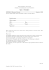Name : DUNEARN SECONDARY SCHOOL End-of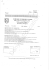Click To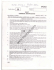I CALCHI - Martini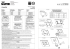Diapositiva 1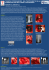ToNELuX oTB16 SoMMAToRE A 16 CANALI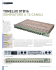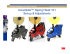cerco amiche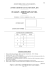La piramide nasale è una delle sedi di più frequente localizzazione